# Gesture Based Visually Writing System Using OpenCV and Python

This is the first part of Gesture Based Visually Writing System.

Contents

## Before Starting

Huge credit goes to the pandemic COVID19 and without it, I would have not been unemployed and the thoughts of writing this blog wouldn’t have appeared. Also the climate change, due to which I usually have blackouts.

## Motivations

We have seen decades of AI revolutions and from Deep Blue chess to Dota playing agents. We are in a competition of achievements. Years ago, there were HOG methods and there was nothing like YOLO or RCNN and the world of Computer Vision today is hungry to find new methods. There are many researchers and academics working continuously to make more AI based systems and the world of Cloud Services has granted huge advantages. The world of Open Source has been so great that we can almost get everything’s code. Now the program can complete our sentences, drawings, can itself talk and many more. The list goes on.

## How come?

I was stuck in a village with no internet and electricity and I was planning on Region-Based method for Corn Infection detection then I started to write using paper and pen. Please follow below links about how I am doing(or will be did?) it. The thought of what if? came. What if we can just move our hand on air and we are actually able to write something? Then rest is on this blog.

I am doing below tasks currently but some unpredicted electricity problems are affecting me.

## Inspiration

This blog post is inspired by the following articles and I have copied some contents also, so I am giving credits to the authors.

• Hand Gesture Recognition using Python and OpenCv The author of this article has made gesture recognition so simple that it made me complete the drawing system within a few hours. Honestly, the part of hand contour extraction is inspired from this blog.

## Prerequisites

For understanding the things going on here, basic Image Processing concepts can work well. For the coding part, understanding of NumPy, Matplotlib and OpenCv will be enough. For NumPy and Matplotlib, you can always checkout this awesome repo of Mr. Tarry Singh. And for OpenCv, documentation is great and don’t forget to checkout PyImagesearch. Also the book Image Operators Image Processing in Python by Jason M. Kinser is a great book to understand Image Processing from scratch. This book takes a guide from basic image formation to advanced topics like segmentation.

## Introduction

Computer Vision has been an area of interest for years. Different competitions have given us new concepts and ideas. Also there has been lots of work on Gesture Recognition and Sign Language Recognition. Gesture Recognition is not an easy task because of the complexity of image formation and the noise on image. The extraction of the hand from the image is another problem. There has been various research to do segmentation of image and one method to do so is selective search(Used on RCNN Family and I am using this method to do Region Proposal on another blog.)

## Problem Statement

We need to extract the hand gesture from the image first and then checkout for the movement of the hand. (For simplicity I am making this blog work for any object movements. But for advanced and more robustness, we must recognize the hand and draw only then.) The problem is so simple that it can be divided onto below steps:-

1. Read the frame from the image and extract the hand region.
2. Draw a pointer on the top of the hand region and draw the pointer on canvas along with the pixels.
3. When the character is drawn on the canvas, send the character to the classification model.

The objective of this blog is to successfully create a Gesture Based Writing System while explaining all major tasks on the way. Also checkout for the bonus topics(there is always some interesting going on).

# Segmentation of Hand Region

For this particular problem, the hand will be the foreground and the other remaining things are the background. Our task will be to find the exact position of the hand. But how can one do that? The simple but tricky concept here is known as Background Subtraction. OpenCV’s fast and powerful functionalities allow us to do this operation in a realistic scenario. The background subtraction is done for each sequence of frames.

# Background Subtraction

The concept of background subtraction is so simple, we take an image which has only a background, then take an image with the same background image. Then subtract those two images, what will you get? Let’s see the code in action.

• First define a good show method.
• And run camera, capture foreground/background images
• Show differences.
def show(img, figsize=(10, 10)):
figure = plt.figure(figsize=figsize)
plt.imshow(img)
plt.xticks([])
plt.yticks([])
plt.show()

cap = cv2.VideoCapture(0) #run camera with device
imgs = [] #list to hold frames
while len(imgs) < 2: #while we captured one foreground and one background image
frame = cv2.flip(frame, 1) #flip the frame for anti-mirror effect
key = cv2.waitKey(2) #wait for 2sec to check user key strike
if key == 32: #if key is space then show the frame, append it to our list
show(frame)
imgs.append(frame)
cv2.imshow('Running Frame', frame) #keep showing our current frame
if key == 27 & 0xFF: #when escape key is pressed, exit
break
cap.release() #release the camera and destroy windows
cv2.destroyAllWindows()
show(imgs-imgs) #background subtraction


The result of this code will not be great but lets see them in the image.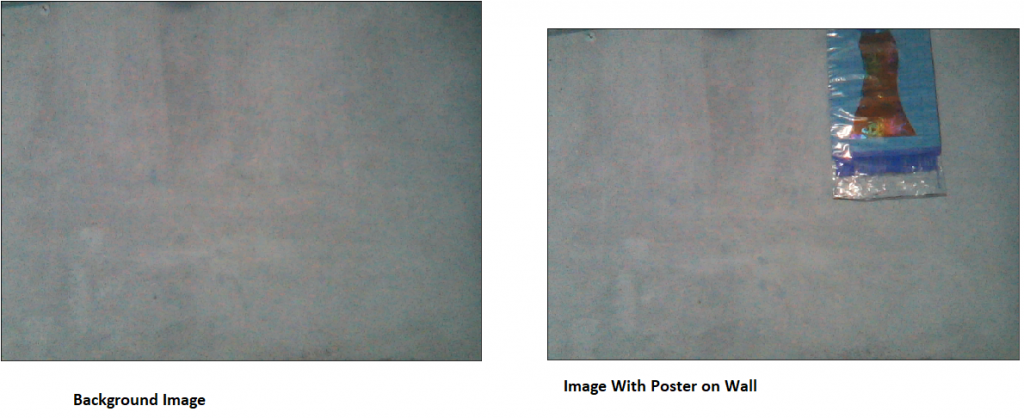The only difference I am using on these images is the pamphlet on the wall. The final difference image doesn’t look like any difference but some noises. This is because of the image quality and noise effect, we can always remove these noise by some factor later. OpenCV reads images in BGR format and it still is on BGR. The difference on the image shows that the object on the wall was not on the background image but is present on the new image hence its color will be different than others and it will be easy to recognize them. Hence the object is foreground and the result will be called the mask.

For better performance, we will use the concept of running average. We will run our system idle for some frames and then take average from it. We will make an average background. Then will do the absolute subtract. Also using RGB/BGR image on background subtraction only increases the complexity, so we will use the Grayscale image or ROI.

The mask is something that holds the foreground. In general, the difference image in the above image also contains some sort of mask on the place where the object is present. The mask is generated by doing absolute subtraction of the current frame with average background and then doing thresholding. The actual mask will contain black on the unwanted region and white on our hand region. This is a pretty simple idea, we are subtracting the background with a background image that contains the hand, the difference will only be on the hand region and all other parts will be black(RGB (0, 0, 0)).

Thresholding is the concept where pixels are grouped into one of two groups by comparing pixels with thresholding values. There are various thresholds, most simple is Binary and others are Otsu, Inverse Binary etc. Let’s see the code on the action.

diff = np.abs(cv2.cvtColor(imgs, cv2.COLOR_BGR2GRAY)-cv2.cvtColor(imgs, cv2.COLOR_BGR2GRAY))
_, th = cv2.threshold(diff, 150, 255, cv2.THRESH_BINARY)
show(th)


Thresholding the difference of gray images will give us a mask. The value will be 0 if it is less than 150 and will be 255 if it is greater than 150. The result is great but not as we expect it to be.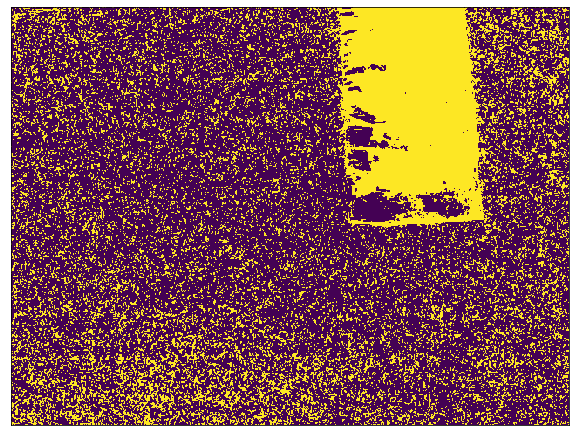The mask is generated for each and every frame(except averaging frames).

# Contour Extraction

The mask on the above image looks weird because it is full of noise and my room is not that lighty. Lesser the noise, the easier the task becomes. The contour is the outer region where an object is located. In our case the contour must be around the hand. But because of the noise, we might have multiple contours and also can we hold our still? Also the shadows can affect. The contour with the largest area is our hand region.

Contour is the outline or bounding curves around the boundary of an object located on an image.

Lets find the contour on above image, can we find the region where the poster is placed?

(cnts, _) = cv2.findContours(th.copy(), cv2.RETR_EXTERNAL, cv2.CHAIN_APPROX_SIMPLE)
segmented = max(cnts, key=cv2.contourArea)
cv2.drawContours(imgs, [segmented], -1, (0, 0, 255))
show(imgs)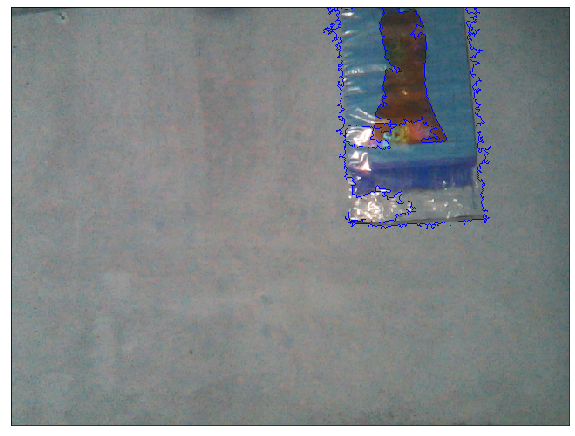Well, so far so good. Please ignore the color channel on this image because I am using BGR color space. But we did a pretty great job or OpenCV did?

# Writing Canvas Generation

The canvas is something where we can write here. So for simplicity, it will be like a whiteboard. But in image processing, it will be black image.

Why black image?

The pixel value of black is [0, 0, 0] so we can do addition without affecting any pixels. The addition here will be what we call writing. Complete black image is like an addition identity image. Thanks to NumPy we can make the complete black image into white just by adding 255.

The basic idea behind writing is to find the top of our contour. Draw a pointer on that position on frame(it will be like we are seeing where our hand is moving). And on the canvas, also make a pointer on the same position, change the color on the place where the pointer is present. The image we will use for operations is black but what we will show is white so we will do some weird operations. Also using OpenCV we can control the camera with our keys, so we can make different modes of pointer. For simplicity, I am making some modes:

• Write:- It will add pixels on the canvas.
• Erase:- It will remove the written pixels from the canvas. It is tricky but works fine. Since our background is white, we will make every pixel white where the pointer resides in this mode.
• Idle:- Neither write nor erase.
• Erase All:- Erases the canvas to make it clean.
• Restart All:- Erases the canvas along with the average image. New Average is taken.

# Implementation

## Background Preparation

We will start by assuming what the background will be for our case. We will do a running average for up to some frames and only then do other processes.

import cv2
import imutils
import numpy as np
import matplotlib.pyplot as plt
def running_average(bg_img, image, aweight):
if bg_img is None:
bg_img = image.copy().astype("float")
else:
cv2.accumulateWeighted(image, bg_img, aweight)
return bg_img


A mathematical formula is:- \begin{equation}\label{eq:} dst(x, y) = (1-\alpha) * dst(x,y) + \alpha * src(x, y) \end{equation}

• dst is destination image or accumulator
• src is source image
• 𝛼 is the weight of the input image and decides the speed of updating. If set small, average is taken over large number of frames.

Here the source is image and the destination is bg_img.

## Preparing Parameters and Variables

aweight = 0.5 #accumulate weight variable
cam = cv2.VideoCapture(0) # strat the device camera
top, right, bottom, left = 250, 400, 480, 640 # ROI box
num_frames=0 # count frame
canvas = None # writing canvas
t=3 # thickness
draw_color = (0, 255, 0) # draw color(ink color)
pointer_color = (255, 0, 0) # pointer color
erase = False # mode flag
take_average=True # flag to indicate take average
bg_img=None #bg image


Please follow the comment written right to the code line for explanation.

## Running the Camera

while True: # loop while everything is true
if ret: # if camera has read frame
key = cv2.waitKey(1) & 0xFF # wait for 1ms to key press
frame = imutils.resize(frame, width=700)
frame = cv2.flip(frame, 1) # flip to remove mirror effect
clone = frame.copy() # clone it to not mess with real frame
h, w = frame.shape[:2]
roi = frame[top:bottom, right:left] # take roi, to send it onto contour/average extraction
gray = cv2.cvtColor(roi, cv2.COLOR_BGR2GRAY) # roi to grayscale
gray = cv2.GaussianBlur(gray, (7, 7), 0) # add GaussianBlur to eliminate some noise


Since we have chosen our device camera from cv2.VideoCapture(0), we will read the frames next. When the camera is not working properly, the ret becomes False. Also we want our system to continuously check for user input, so we will do cv2.waitKey(1) & 0xFF. The original frames will have to be flipped in order to make it realistic. We can do that by flipping on the x-axis using cv2.flip(frame, 1). Then we crop the ROI from the given box and convert it into Grayscale. Grayscale is much more efficient at doing thresholding processes. Then we added some Gaussian blur of shape (7, 7). Some kind of Convolution Operation happens inside that function. If you want to learn about Convolution operation from Scratch then follow the link below.

## Take Average of ROI Background

        if num_frames < 100 and take_average == True: # if to take average and num frames on average taking is lesser
bg_img = running_average(bg_img, gray, aweight) # perform running average
cv2.putText(clone, str(num_frames), (100, 100),
cv2.FONT_HERSHEY_SIMPLEX, 0.5, (0, 0, 255), 5) # put frame number on frame
num_frames += 1
else: # if not to take average
num_frames = 0
hand = get_contours(bg_img, gray) # take our segmented hand
take_average=False
cv2.rectangle(clone, (left, top), (right, bottom), (0, 255, 0), 2) # draw a ROI
cv2.imshow("Feed", clone) # show live feed
if key==27: # if pressed  escape, loop out and stop processing
break
cam.release()
cv2.destroyAllWindows()


Next, we check the flag if we had to take average or not, if we do want to find average still, then put frame number on the frame. Else we get contours from our image. Finally, we draw a rectangle as ROI on live feed and if the user enters the escape key then stops the process.

## Get Hand Contours

Next we do find our contours on the current frame, this is done by taking the absolute difference between average background image and current frame, then doing thresholding.

def get_contours(bg_img, image, threshold=10):
diff = cv2.absdiff(bg_img.astype("uint8"), image) # abs diff betn img and bg
_, th = cv2.threshold(diff, threshold, 255, cv2.THRESH_BINARY)
(cnts, _) = cv2.findContours(th.copy(), cv2.RETR_EXTERNAL, cv2.CHAIN_APPROX_SIMPLE)
if len(cnts) == 0:
return None
else:
max_cnt = max(cnts, key=cv2.contourArea)
return th, max_cnt


The function takes the background image, current frame and the thresholding value. It returns the thresholded image and the largest contour.

## Drawing Contours and Showing Thresholded image

    ........................
........................
else: # if not to take average
num_frames = 0
hand = get_contours(bg_img, gray) # take our segmented hand
take_average=False
if hand is not None:
thresholded, segmented = hand
cv2.drawContours(clone, [segmented+(right,top)], -1, (0, 0, 255))


The contours returned are from the ROI image which is the cropped version of the original frame. Hence we have to add the starting position of the ROI box to correctly draw contours on the original frame. I hope the image just looks like the one below’s.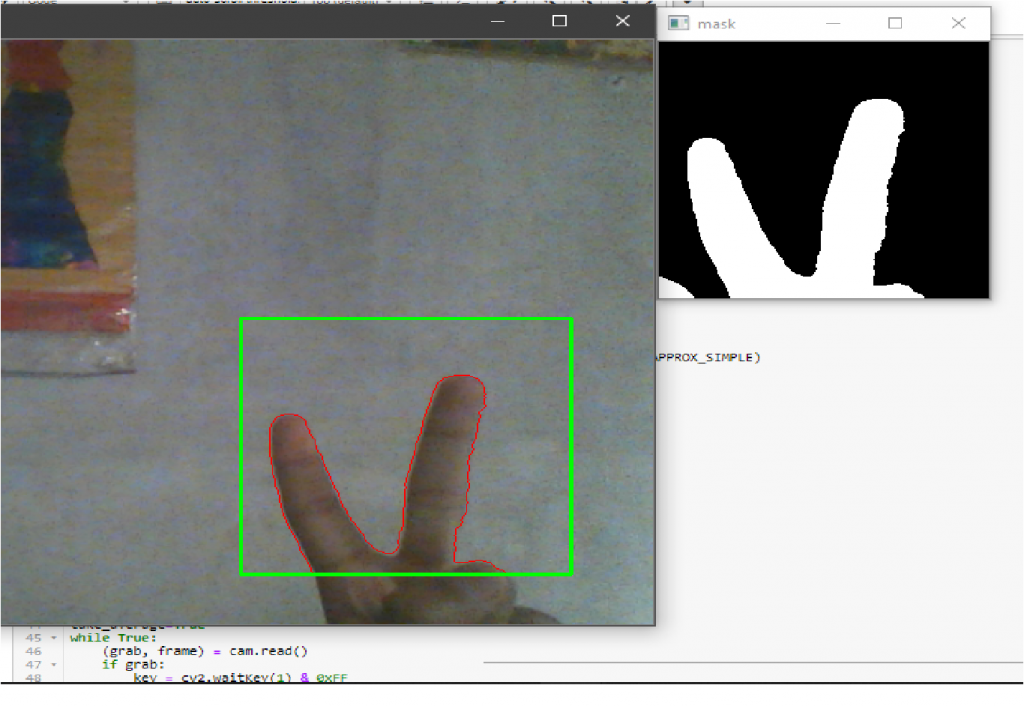## Draw Pointer on Canvas

    .......................
.......................
else: # if not to take average
num_frames = 0
hand = get_contours(bg_img, gray) # take our segmented hand
take_average=False
if hand is not None:
thresholded, segmented = hand
cv2.drawContours(clone, [segmented+(right,top)], -1, (0, 0, 255))
tshape = thresholded.shape
sshape = segmented.shape
new_segmented = segmented.reshape(sshape, sshape[-1])
m = new_segmented.min(axis=0)
if type(canvas) == type(None):
canvas = np.zeros((tshape, tshape, 3))+255
c = np.zeros(canvas.shape, dtype=np.uint8)
cv2.circle(c, (m, m), 5, pointer_color, -3)
cv2.drawContours(clone, [segmented+(right,top)], -1, (0, 0, 255))
cv2.circle(clone, (right+m, top+m), 5, pointer_color, -3)


We want to draw a pointer only inside the ROI box and not outside. And also the contours are found on the cropped ROI image hence we need to add right and top values. The writing canvas is first initialized with zeros and then added 255, thanks to NumPy array broadcasting, hence canvas became completely white. The variable c here is a very important one and it is responsible to show the pointer. The pointer window is equal to the canvas, and the pointer image is 0 on every place except where the minimum position of contour is.

### Lets recap:-

• The frame is read and the contours are calculated by taking the absolute difference of current frame with the average background image.
• The contour is drawn on live feed.
• Threshold image is shown.
• The pointer(circle) is drawn on live feed.
• The pointer image, canvas image is prepared.

The pointer should move along with fingers. Please check the code below if your system is not running well.

def running_average(bg_img, image, aweight):
if bg_img is None:
bg_img = image.copy().astype("float")
else:
cv2.accumulateWeighted(image, bg_img, aweight)
return bg_img
def get_contours(bg_img, image, threshold=10):
diff = cv2.absdiff(bg_img.astype("uint8"), image)    # abs diff betn img and bg
_, th = cv2.threshold(diff, threshold, 255, cv2.THRESH_BINARY)
(cnts, _) = cv2.findContours(th.copy(), cv2.RETR_EXTERNAL, cv2.CHAIN_APPROX_SIMPLE)
if len(cnts) == 0:
return None
else:
max_cnt = max(cnts, key=cv2.contourArea)
return th, max_cnt
aweight = 0.5 #accumulate weight variable
cam = cv2.VideoCapture(0) # strat the camera
top, right, bottom, left = 250, 400, 480, 640 # ROI box
num_frames=0 # count frame
canvas = None # writing canvas
t=3 # thickness
draw_color = (0, 255, 0) # draw color(ink color)
pointer_color = (255, 0, 0) # pointer color
erase = False # mode flag
take_average=True # flag to indicate take average
bg_img=None #bg image
while True: # loop while everything is true
if ret: # if camera has read frame
key = cv2.waitKey(1) & 0xFF # wait for 1ms to key press
frame = imutils.resize(frame, width=700)
frame = cv2.flip(frame, 1) # flip to remove mirror effect
clone = frame.copy() # clone it to not mess with real frame
h, w = frame.shape[:2]
roi = frame[top:bottom, right:left] # take roi, to send it onto contour/average extraction
gray = cv2.cvtColor(roi, cv2.COLOR_BGR2GRAY)  # roi to grayscale
gray = cv2.GaussianBlur(gray, (7, 7), 0) # add GaussianBlur to eliminate some noise

if num_frames < 100 and take_average == True: # if to take average and num frames on average taking is lesser
bg_img = running_average(bg_img, gray, aweight) # perform running average
cv2.putText(clone, str(num_frames), (100, 100),
cv2.FONT_HERSHEY_SIMPLEX, 0.5, (0, 0, 255), 5) # put frame number on frame
num_frames += 1
else: # if not to take average
num_frames=0
hand = get_contours(bg_img, gray)  # take our segmented hand
take_average=False
if hand is not None:
thresholded, segmented = hand
cv2.drawContours(clone, [segmented+(right,top)], -1, (0, 0, 255))
tshape = thresholded.shape
sshape = segmented.shape
new_segmented = segmented.reshape(sshape, sshape[-1])
m = new_segmented.min(axis=0)

if type(canvas) == type(None):
canvas = np.zeros((tshape, tshape, 3))+255
c = np.zeros(canvas.shape, dtype=np.uint8)
cv2.circle(c, (m, m), 5, pointer_color, -3)
cv2.circle(clone, (right+m, top+m), 5, pointer_color, -3)
cv2.imshow("c", c)
cv2.rectangle(clone, (left, top), (right, bottom), (0, 255, 0), 2) # draw a ROI
cv2.imshow("Feed", clone) # show live feed
if key == 32:
cv2.imwrite("Captured.png", clone)
if key==27: # if pressed  escape, loop out and stop processing
break
cam.release()
cv2.destroyAllWindows()


## Draw on Canvas

                ...................................................
...................................................
cv2.circle(c, (m, m), 5, pointer_color, -3)
cv2.circle(clone, (right+m, top+m), 5, pointer_color, -3)
cv2.imshow("c", c)
cv2.circle(canvas, (m, m), 5, draw_color, -3)
e = cv2.erode(canvas, (3, 3), iterations=5)
drawn = cv2.resize(e, (clone.shape, clone.shape))
c = cv2.resize(c, (clone.shape, clone.shape))
drawn_new = drawn+c
cv2.imshow("Drawing", drawn+c)


What is happening here?

Well, on canvas, in the position where the minimum position of contour is present, we draw a circle of given thickness with a different color than the pointer. The canvas, pointer, thresholded, ROI image all will be of the same size but we want it to be just the same as our live feed, hence we resize it. But why do erosion? Erosion is used here to make the image less noisy or more thick around the drawn circle. The canvas here is an original canvas. And we don’t do anything other than write on it. But we resize the erosion result. Then to show the drawn color and pointer at the same time on canvas, we will sum the canvas with the pointer image. The beauty of black image summing with any image is so awesome here.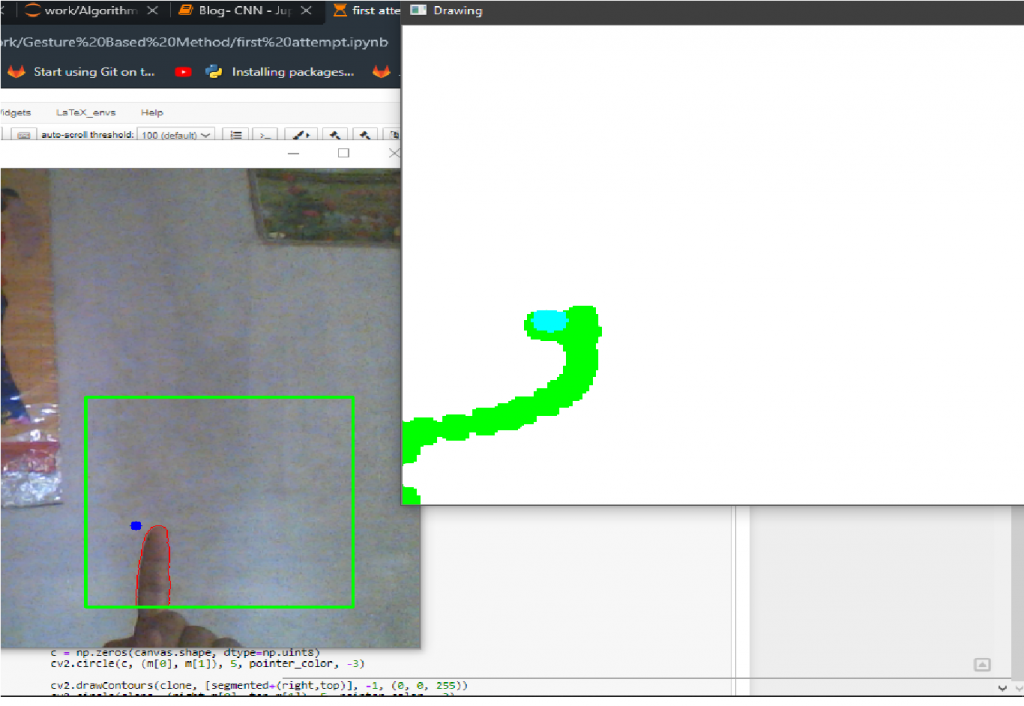Befor going onto modes, lets first define our keys to do modes and other operations.

• escape:- Exit from system.
• space:- Save current drawing(excluding pointer).
• z:- Idle mode(move only pointer).
• x:- Erase mode.
• c:- Color mode.
• e:- Erase current canvas but leave the average image.
• r:- Restart the average and canvas.

Let’s make basic assumptions:-

• If we want to erase, then the position where the current pointer lies must be made white on canvas.
• If we want to move, then the position where the current pointer lies must be left unchanged on canvas.
• Also make the pointer color change on every mode.

## Preparing Keys

            ...........................
..........................
if hand is not None:
if chr(key) == "x": # if pressed x, erase
draw_color = (255, 255, 255)
pointer_color = (0, 0, 255)
erase = True
if chr(key) == "c":
draw_color = (0, 255, 0)
pointer_color = (255, 0, 0)
erase = False
if chr(key) == "z": #idle
erase = None
pointer_color = (0, 0, 0)
if chr(key) == "r": # restart system
take_average=True
canvas = None
if chr(key) == "e":
canvas = None
drawn = np.zeros(drawn.shape)+255


Above, we are using the flag erase, to do 3 tasks. The pointer color must also be changed per mode.

## Erase mode

                ..........................................
...........................................
c = np.zeros(canvas.shape, dtype=np.uint8)
cv2.circle(c, (m, m), 5, pointer_color, -3)
cv2.circle(clone, (right+m, top+m), 5, pointer_color, -3)
if erase==True:
cv2.circle(canvas, (m, m), 5, draw_color, -3)
erimg=cv2.circle(canvas.copy(), (m, m), 5, (0, 0, 0), -3)
cv2.circle(c, (m, m), 5, (0, 0, 255), -3)
e = cv2.erode(erimg, (3, 3), iterations=5)
drawn = cv2.resize(e, (clone.shape, clone.shape))
c = cv2.resize(c, (clone.shape, clone.shape))
drawn_new = drawn+c
cv2.imshow("Drawing", drawn+c)
erimg=cv2.circle(canvas.copy(), (m, m), 5, (255, 255, 255), -3)
cv2.circle(c, (m, m), 5, (0, 0, 255), -3)
e = cv2.erode(erimg, (3, 3), iterations=5)
drawn = cv2.resize(e, (clone.shape, clone.shape))


I have scratched my head for hours to make this work right. I hope somebody finds a better way to do this.

• First draw a white color on the pointer position on canvas.
• Draw a black color on the pointer position on a copy of the current canvas.
• Take copy image and erode it, resize it and sum it with pointer image.(if the black color is not used then pointer will not be visible)
• And undo the color operation else the pointer position remains on new images.

## Idle Mode

                .....................................................
.....................................................
if erase==True:
cv2.circle(canvas, (m, m), 5, draw_color, -3)
erimg=cv2.circle(canvas.copy(), (m, m), 5, (0, 0, 0), -3)
cv2.circle(c, (m, m), 5, (0, 0, 255), -3)
e = cv2.erode(erimg, (3, 3), iterations=5)
drawn = cv2.resize(e, (clone.shape, clone.shape))
c = cv2.resize(c, (clone.shape, clone.shape))
drawn_new = drawn+c
cv2.imshow("Drawing", drawn+c)
erimg=cv2.circle(canvas.copy(), (m, m), 5, (255, 255, 255), -3)
cv2.circle(c, (m, m), 5, (0, 0, 255), -3)
e = cv2.erode(erimg, (3, 3), iterations=5)
drawn = cv2.resize(e, (clone.shape, clone.shape))
elif erase==False:
cv2.circle(canvas, (m, m), 5, draw_color, -3)
e = cv2.erode(canvas, (3, 3), iterations=5)
drawn = cv2.resize(e, (clone.shape, clone.shape))
c = cv2.resize(c, (clone.shape, clone.shape))
drawn_new = drawn+c
cv2.imshow("Drawing", drawn+c)
elif erase == None:
canvas_shape = canvas.shape
clone_shape = clone.shape
eshape = (clone_shape/canvas_shape, clone_shape/canvas_shape)
m = int(eshape*m)
m = int(eshape*m)
drawn = cv2.resize(drawn, (clone.shape, clone.shape))
dc = drawn.copy()
cv2.circle(dc, (m, m), 10, pointer_color, -3)
cv2.imshow("Drawing", dc)

• When x key is pressed, erase is true and the first condition runs.
• When c key is pressed, erase is fale and the second condition runs.
• When z key is pressed, erase is None and the 3rd condition runs.

In Idle mode, we take the previous drawn image, which is the resized version. We also did some resizing there. In a simple way, we take the copy of the current version of the canvas and draw a pointer above it just to show movement-like features. The showing of movement is just a fooling idea. In fact this overall operation is a fooling idea.

## Restart mode

When the key r is pressed, take_average becomes true and the averaging strats.

## Erase All mode

When the key e is pressed, drawn is made white. And the canvas is restarted.

# Bonus Part

I am going to do Character Recognition on the characters written on the canvas. For that, I will be using the Devanagari Handwritten dataset. For simple Devanagari Handwritten characters, I have written a Detection/Localization or OCR like system years ago using NumPy, Keras and OpenCv. Please follow the below blog post for full contents. The codes and algorithms are not good and I have not got time to improve it. But I used imagination on the coding so years after I still feel proud of that work.

On that repository, contains a file recognition.py, which is responsible for lots of things. So, here I am also using the same file, and it contains the module recognition inside it. Importantly, I am naming that folder as dcr. While doing some work, I am encountering some errors. Those errors are newbie errors telling about not finding saved model directory. Looking into the predictor.py file and doing some path correction solves the problem. In my case, I did:-

.......................
.......................
def prediction(img):
curr_dir = os.getcwd()+"/dcr/"
json_file = open(curr_dir+'cnn2/cnn2.json', 'r')
json_file.close()
..................................
..................................


Next let our system fire recognition while pressing s key.

...................................
...................................
cv2.imshow("Drawing", dc)
if chr(key) == "s":
show(drawn)
d = drawn.copy().astype(np.uint8)
r = recognition(cv2.cvtColor(d, cv2.COLOR_BGR2GRAY), 'show')
cv2.rectangle(clone, (left, top), (right, bottom), (0, 255, 0), 2) # draw a ROI
cv2.imshow("Feed", clone) # show live feed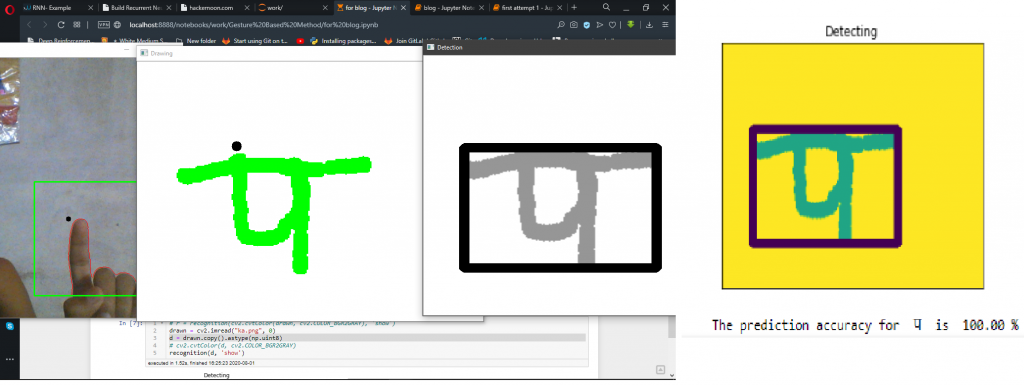The problem with a combination of DCR(Devanagari Character Recognition) and Gesture Writing is only that it doesn’t quite work with words. The reason is the thickness of our writing. So in near future, I will work on that.

# Where From Here?

• What about combining this with some LSTMS or RNN models to predict what is user’s next draw might be?
• Adding a GUI like functionalities where we do hand extraction and then whenever we click on a particular region on frame then fire some actions. Like when we show some GUI like area on camera, when a hand is taken above that area then we fire some events, like changing a color, drawing a line or playing a music etc.

Leave me a comment for the entire codes.

Tags:

Categories:

Updated: